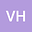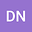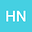A survey on random fractional differential equations involving the generalized Caputo fractional-order derivative
•••Dinh Phu Nguyen
Faculty of Engineering Technology, Quang Trung University, Vietnam
Author ProfileHoa Ngo
Ton Duc Thang University
Author Profile## Abstract

The main content of this paper is focusing on introducing a general form of random fractional differential equations (RFDEs) with the concept of a Caputo-type fractional derivative with respect to another function. The problem proposed here allows us to interpolate various types of RFDEs. By applying the fixed point theorem, the results of the existence and uniqueness of a solution of RFDE are presented. Furthermore, we also propose a new technique called the Hybrid Laplace-like transform method (HLTM) to solve the solutions of RFDEs in the nonlinear form. To visualize the theoretical results, some examples and numerical simulations are provided.